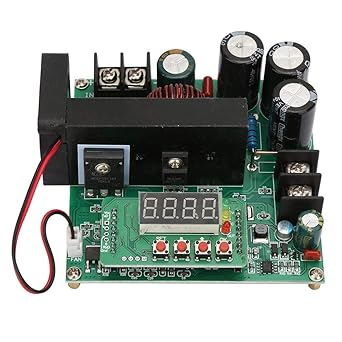# Constant voltage or constant current: which are the differences

Categories : Lighting

In the world of electronics, there are two common ways to power a circuit: constant voltage and constant current. While both can power an electronic circuit, there are some important differences between the two and in this article, we will explore them.## What is the constant voltage

Constant voltage is a power mode in which a fixed voltage is supplied to the circuit, regardless of the load. In other words, the voltage remains constant, but the current flowing through the circuit varies based on the resistance of the load. The current flowing through the circuit is determined by Ohm's law, which states that current is proportional to voltage and inversely proportional to the resistance of the load.

## What is the constant current

In contrast, constant current is a power mode in which a fixed current is supplied to the circuit, regardless of the load. In other words, the current remains constant, but the voltage that is supplied to the load varies based on the resistance. The voltage that is supplied to the circuit is determined by Ohm's law, which states that the voltage is proportional to the current and the resistance of the load.

## Differences between constant voltage and constant currentPrecisely for this reason one of the main differences between constant voltage and constant current is the way in which the load is managed. With constant voltage, the load can vary, and the current through the circuit will vary accordingly. This means that circuits powered at a constant voltage are ideal for loads that can vary significantly. However, if the load becomes too large, the voltage supplied may not be sufficient to power the circuit.

With constant current however, the load can only vary to a certain extent, as the voltage supplied to the circuit will vary based on the resistance of the load. This means that constant current powered circuits are ideal for loads that have a known, constant resistance. If the resistance of the load varies too much, the voltage supplied may be insufficient to power the circuit.

Another difference between constant voltage and constant current is their application in different situations. Constant voltage is commonly used in circuits where it is necessary to control the voltage supplied to the load, such as in the power circuits of electronic devices.

On the other hand, constant current is commonly used in circuits where it is necessary to control the current supplied to the load, such as in LED power circuits.

In addition to these differences, there are a few other considerations that must be made when choosing between constant voltage and constant current. For example, constant voltage can be more energy efficient than constant current. This is because constant voltage allows you to reduce power consumption more efficiently when the load is small. Also, constant voltage is easier to control and regulate than constant current.

On the other hand, constant current has some specific applications where it is preferable over constant voltage. For example, in LED power circuits, constant current ensures constant and uniform illumination of the LEDs, improving the energy efficiency of the circuit and extending the life of the LEDs.

In general, the choice between constant voltage and constant current depends on the specific needs of the electronic circuit and the load it has to supply. It is important to evaluate the load resistance and energy efficiency of the circuit to determine which power mode is most appropriate.

In summary, the differences between constant voltage and constant current are in how the load is handled, the application in different situations and their energy efficiency. Constant voltage is ideal for loads that can fluctuate significantly, such as in power circuits for electronic devices, while constant current is preferred in power circuits for LEDs and other applications where it is necessary to control the current supplied to the load .

Let's find out more on our Diffusione Luce website!

Written by Alice Pruccoli